# R S Aggarwal Solutions for Class 11 Maths Chapter 27 Limits Exercise 27A

R S Aggarwal Solutions for Class 11 Maths are given here for Chapter 27 exercise 27A. This exercise of RS Aggarwal Solutions is based on fundamental theorems on limits and rules for finding function’s limit. This study material contains solved exercises with explanations given by BYJU’S experts. Exercise 27A also gives a brief overview on limits definitions and some important formulas. Students can download R S Aggarwal class 11 solutions pdf for free and start practising.

## Download PDF of R S Aggarwal Solutions for Class 11 Maths Chapter 27 Limits Exercise 27A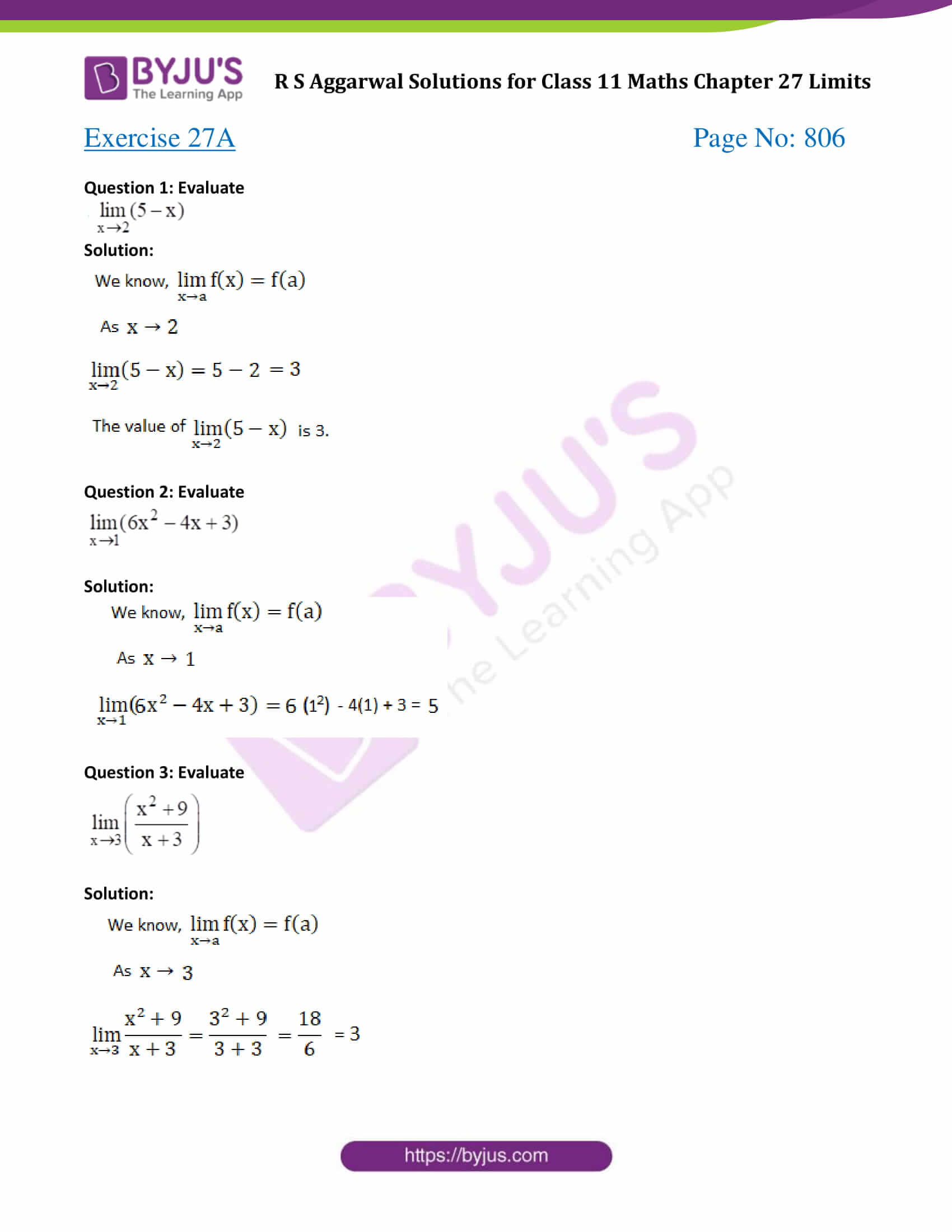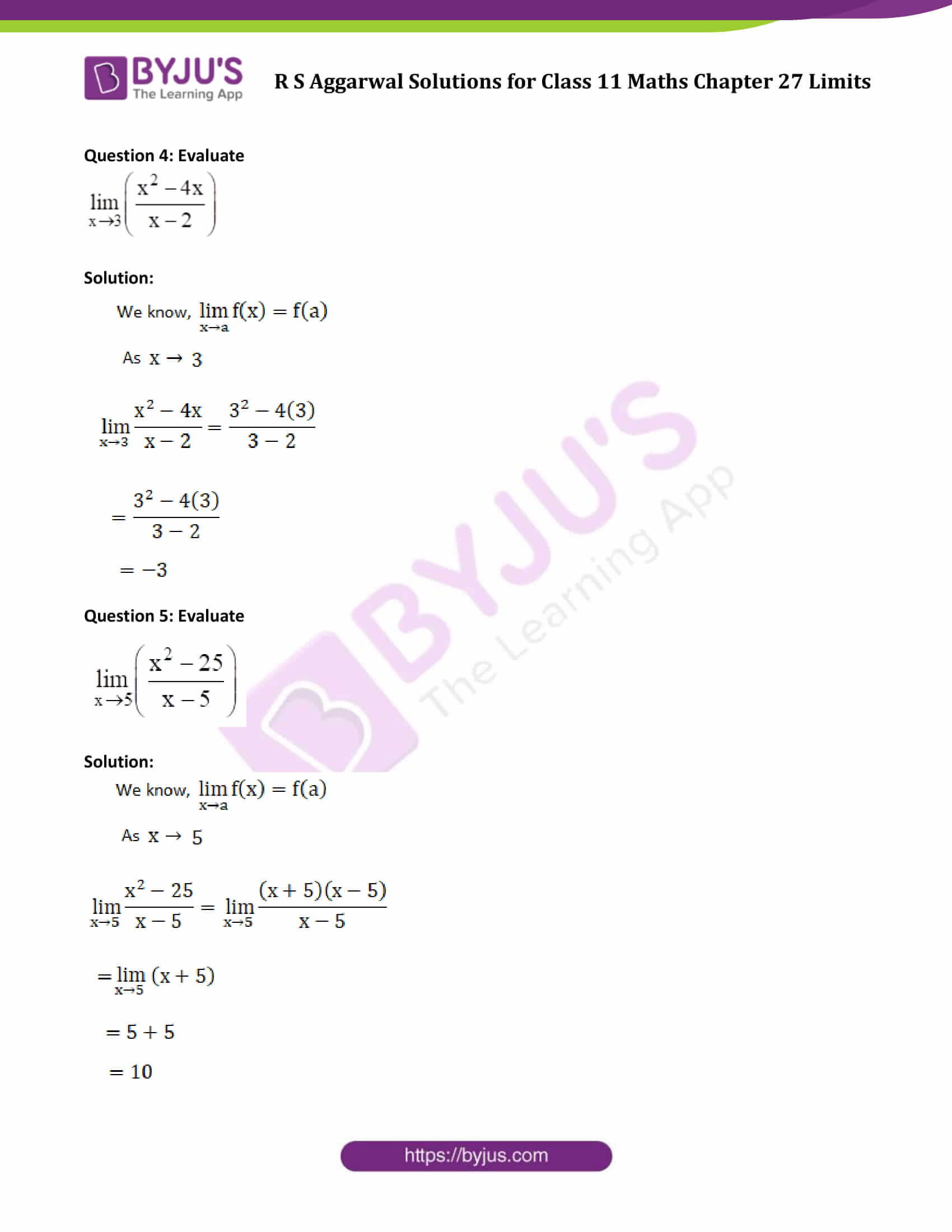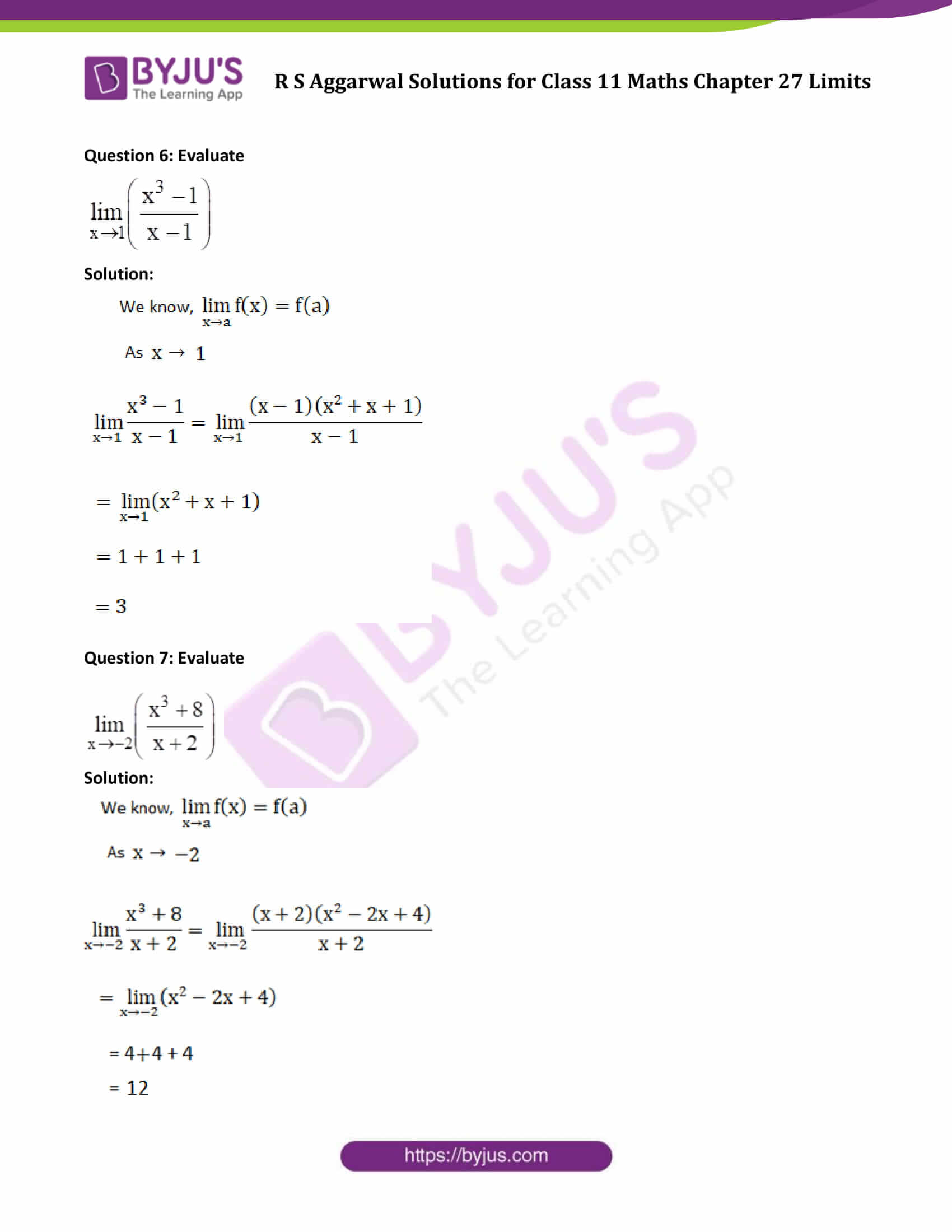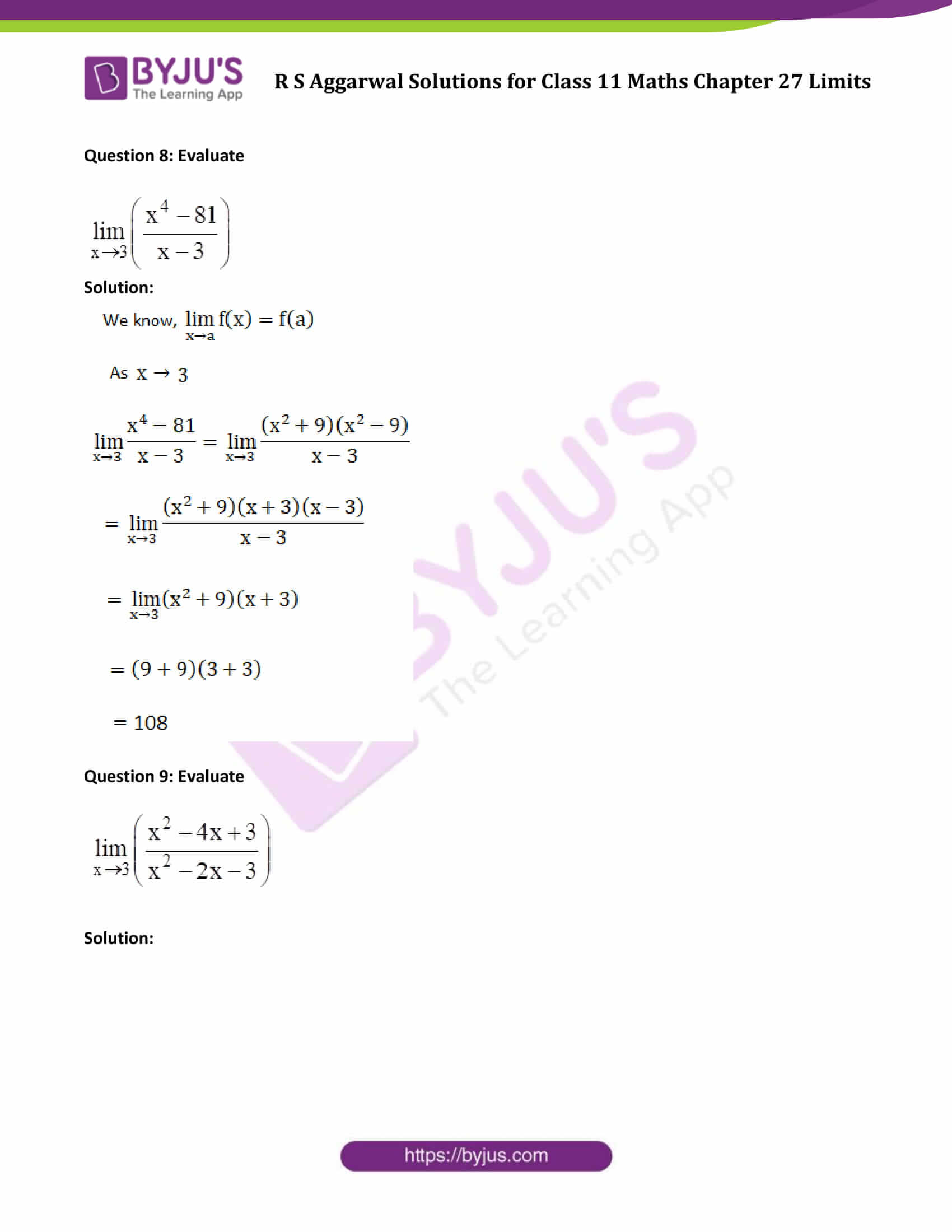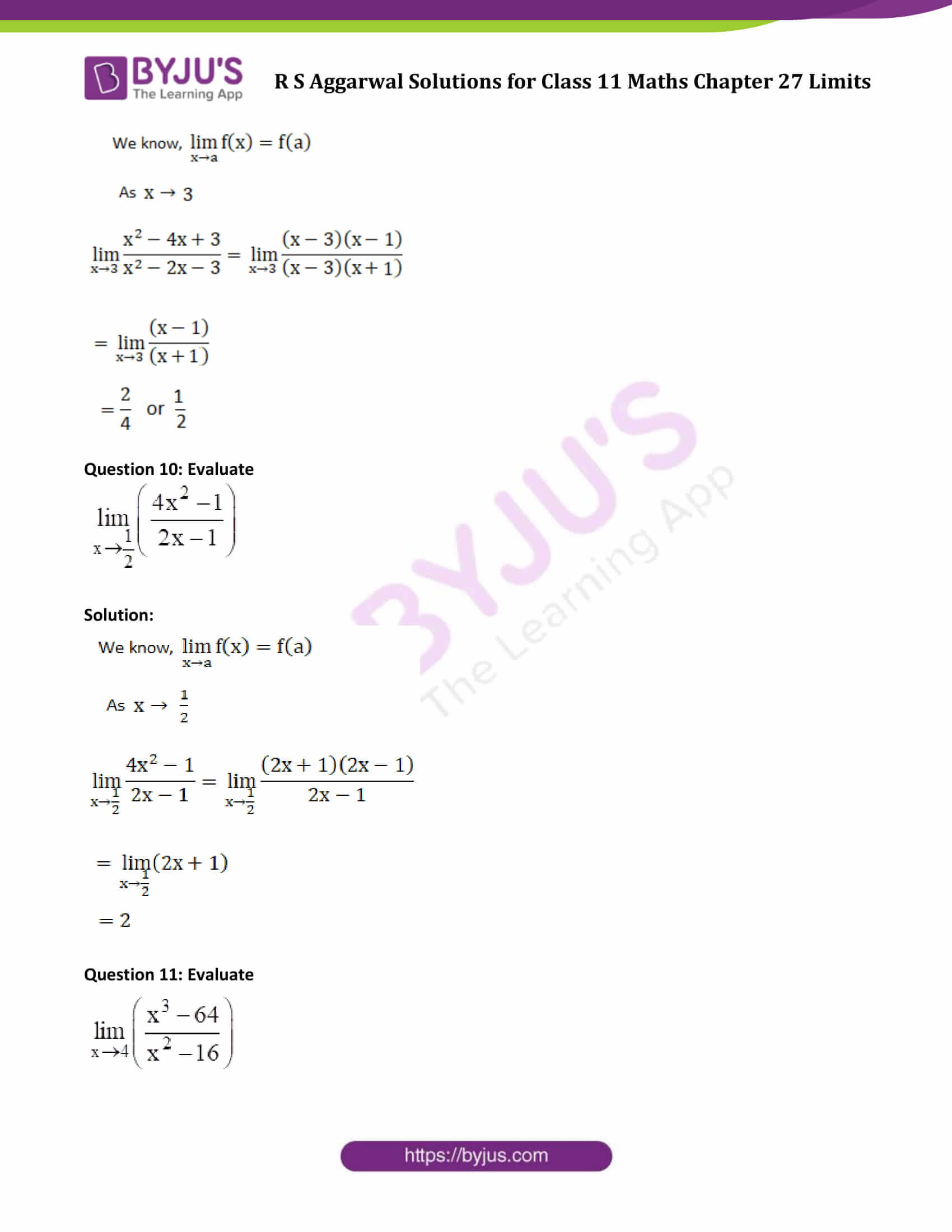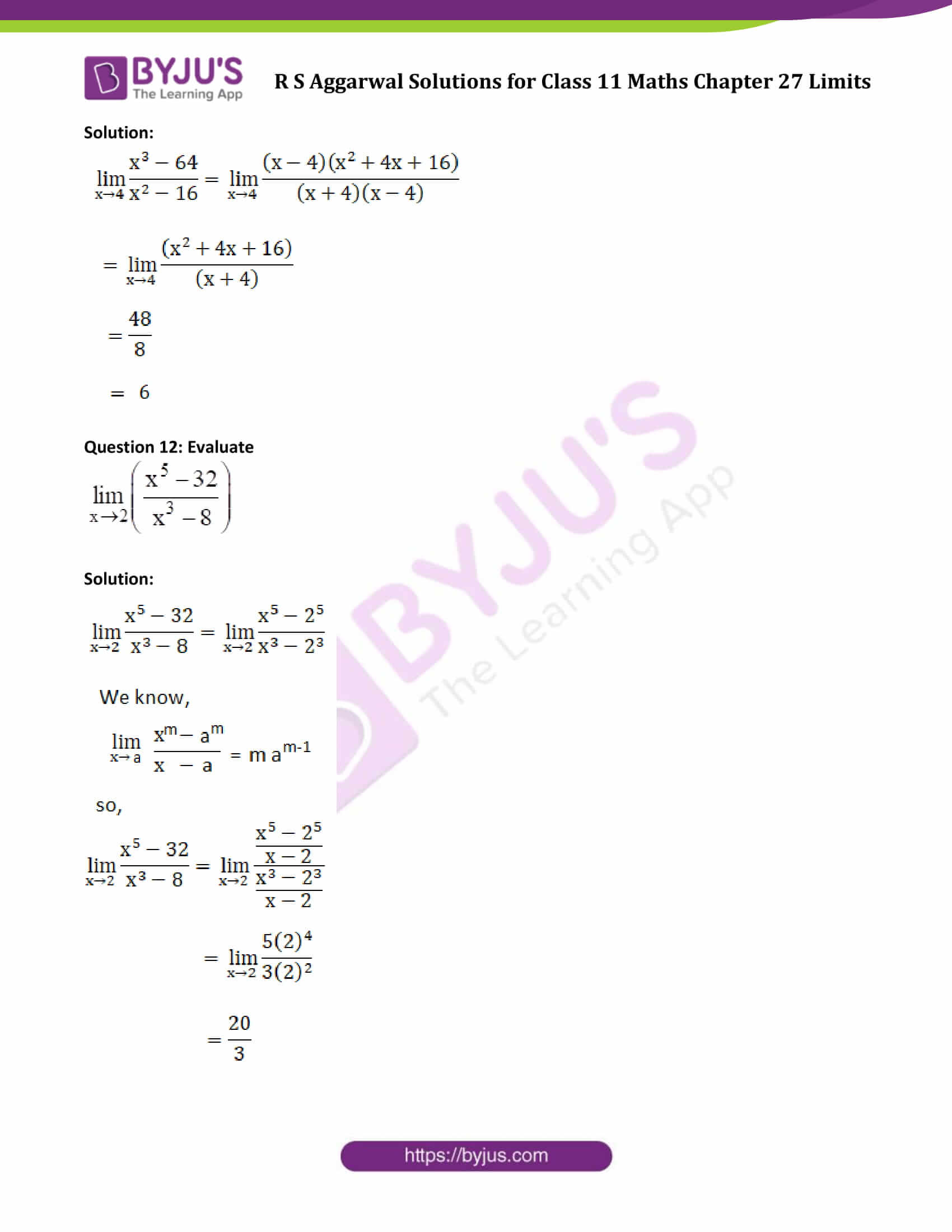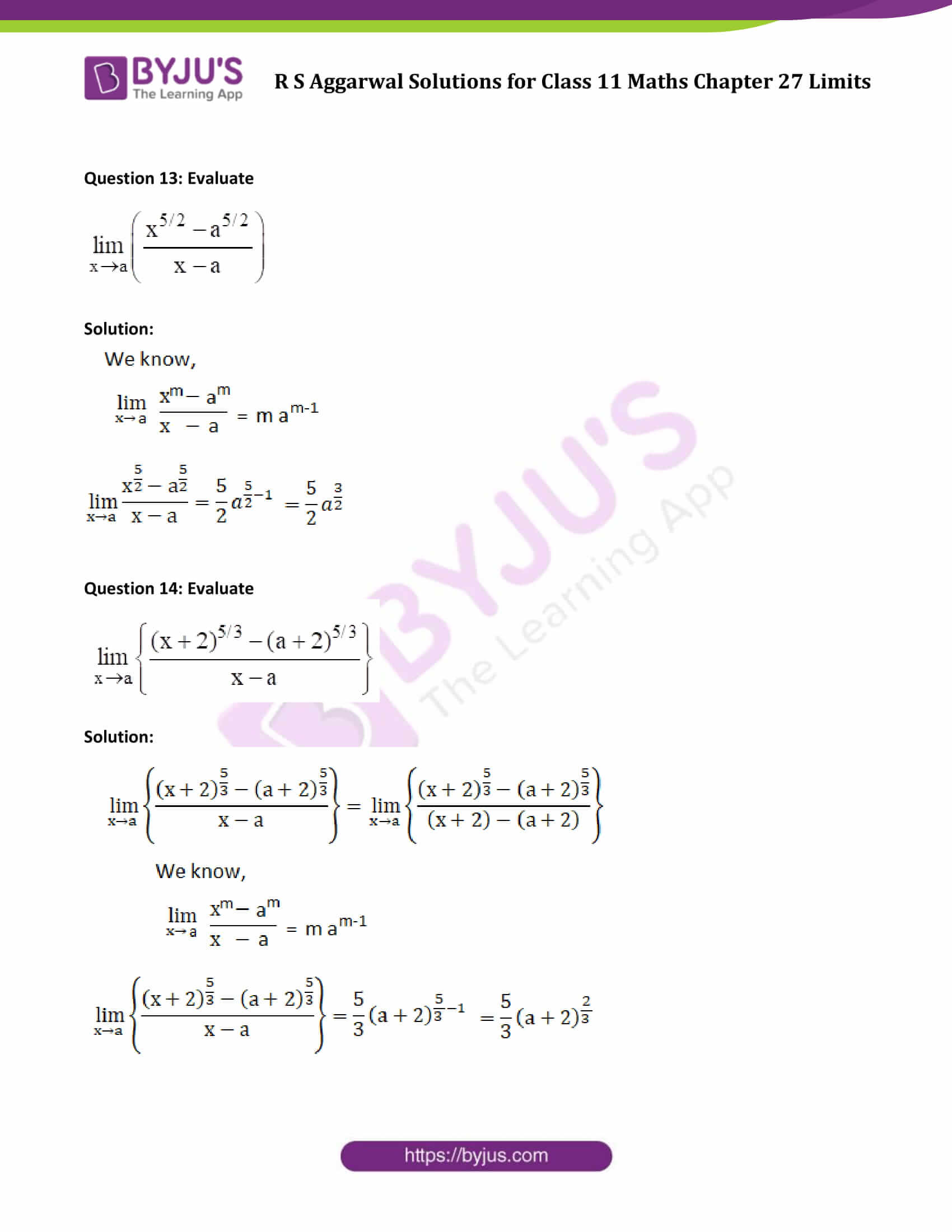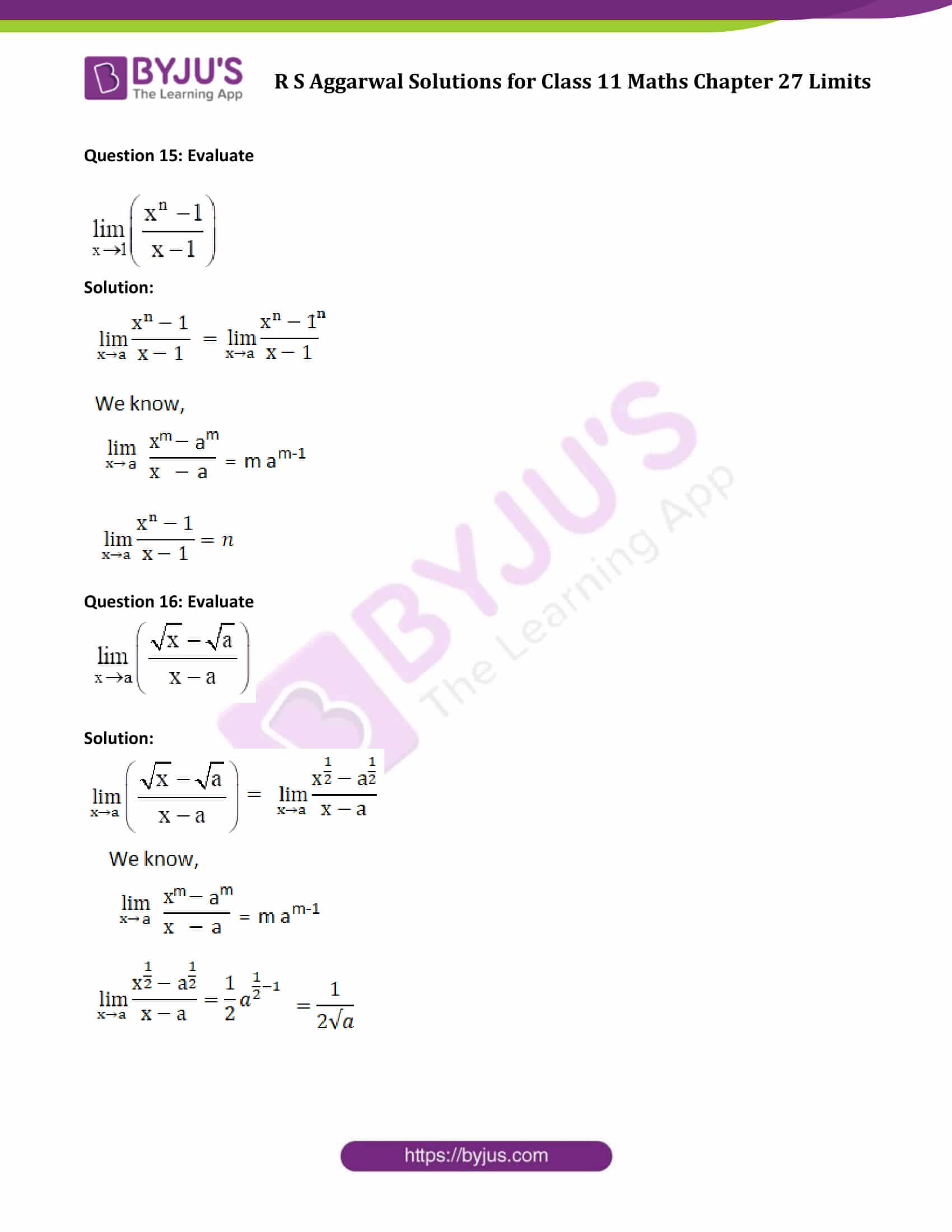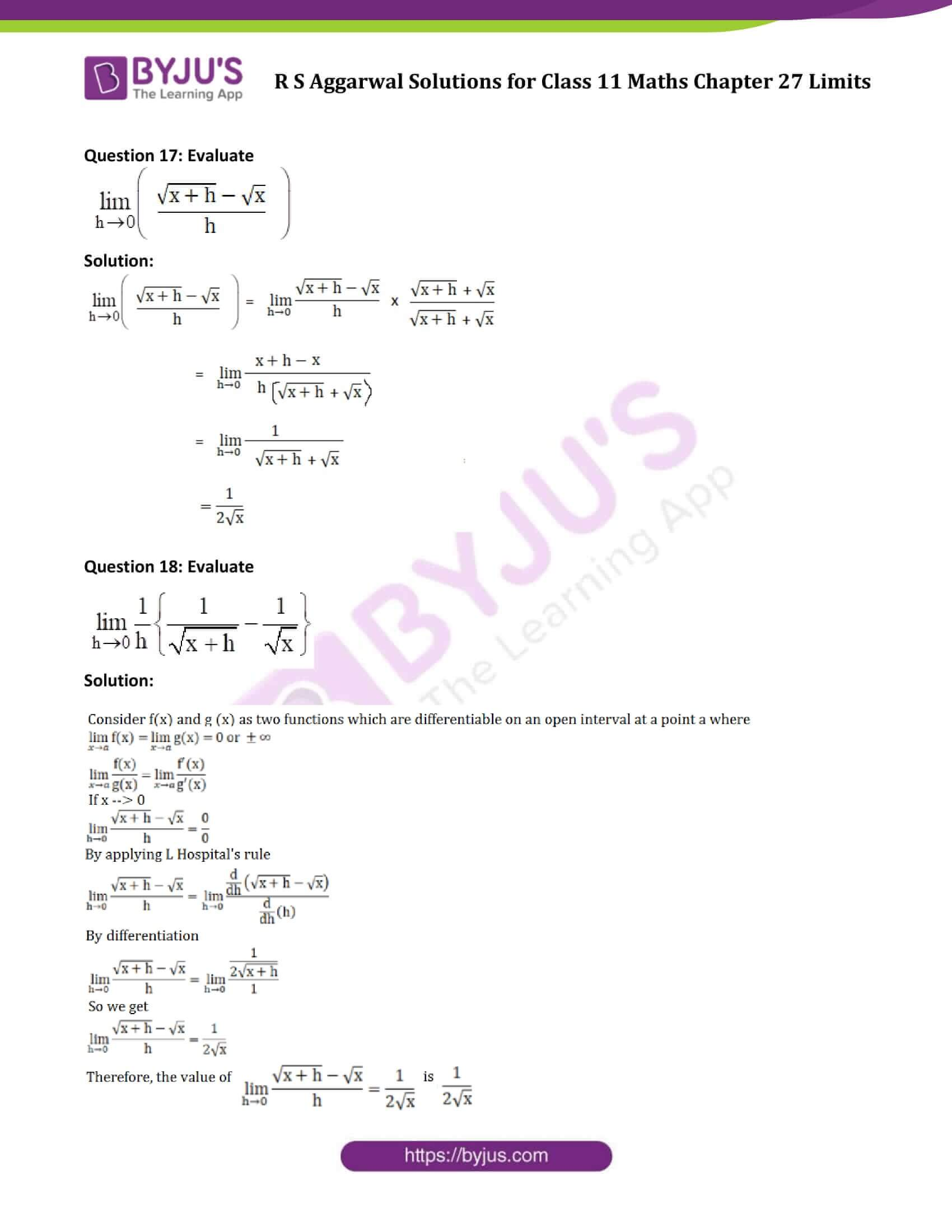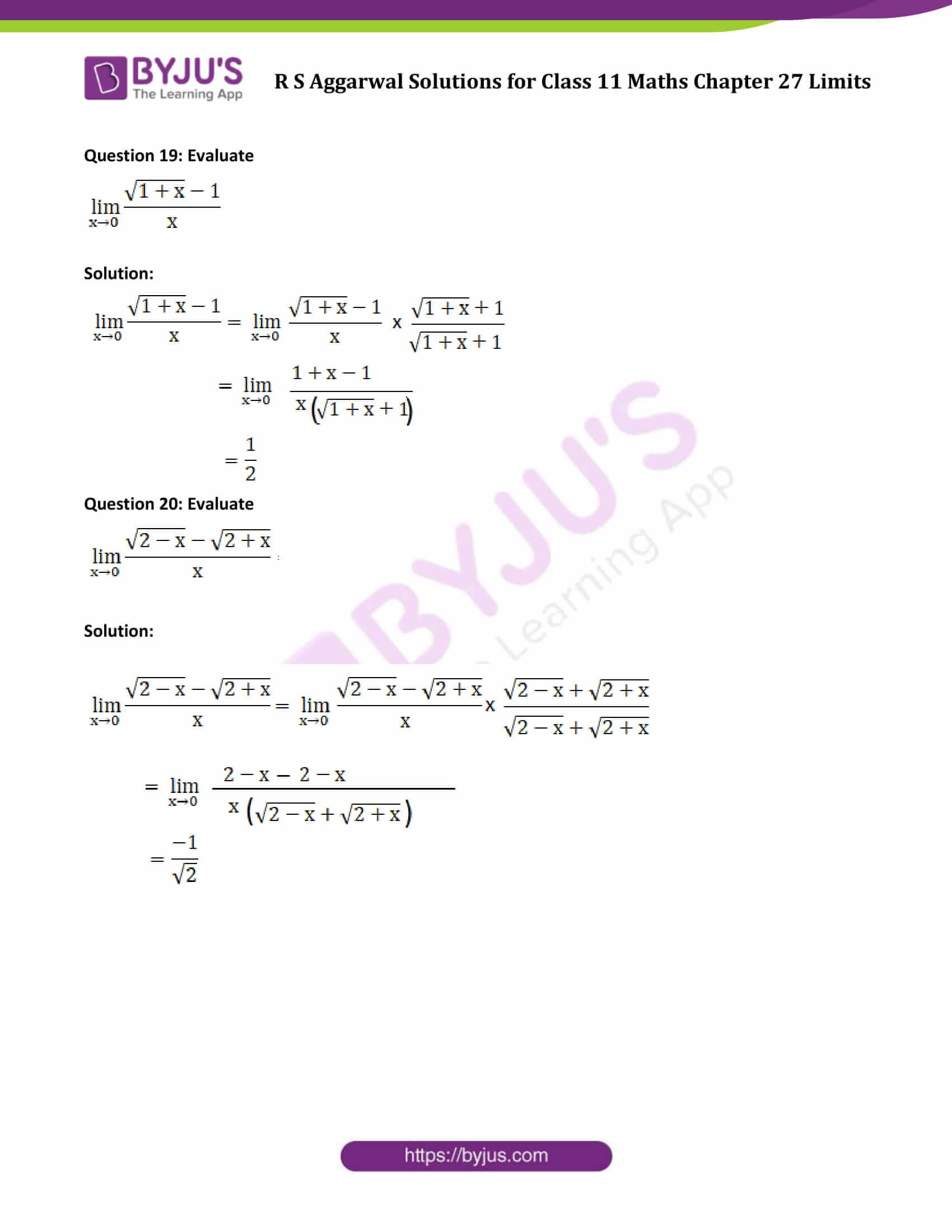### Access Answers of R S Aggarwal Solutions for Class 11 Maths Chapter 27 Limits Exercise 27A Page number 806

Question 1: Evaluate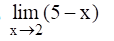Solution: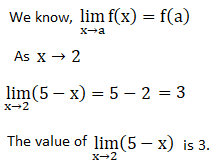Question 2: Evaluate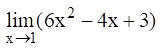Solution: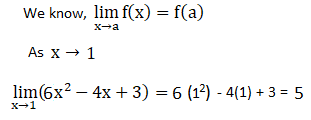Question 3: Evaluate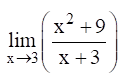Solution: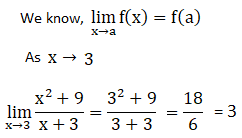Question 4: Evaluate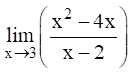Solution: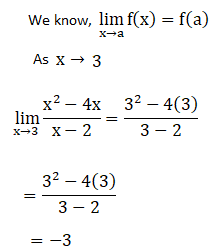Question 5: Evaluate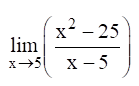Solution: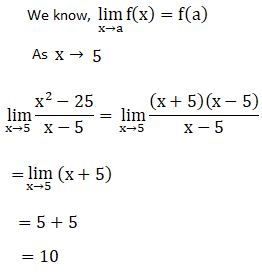Question 6: Evaluate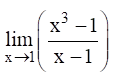Solution: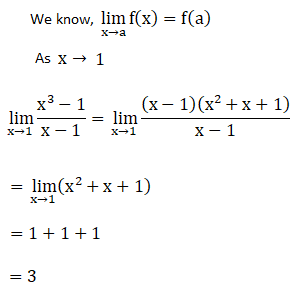Question 7: Evaluate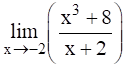Solution: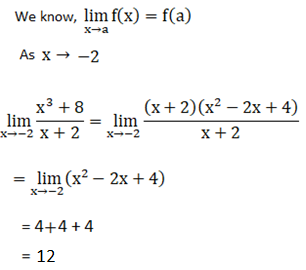Question 8: Evaluate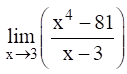Solution: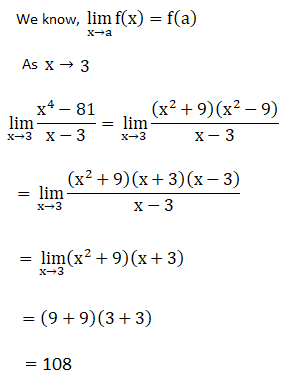Question 9: Evaluate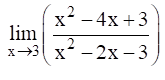Solution: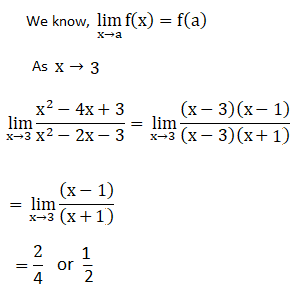Question 10: Evaluate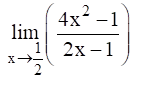Solution: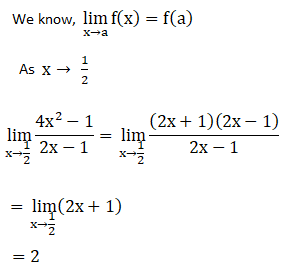Question 11: Evaluate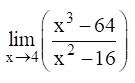Solution: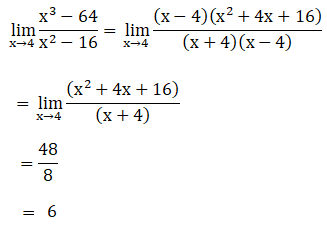Question 12: Evaluate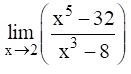Solution: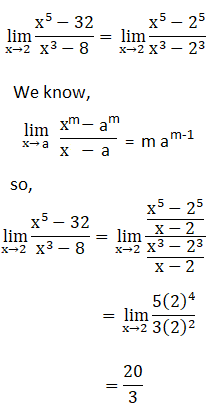Question 13: Evaluate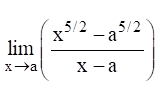Solution: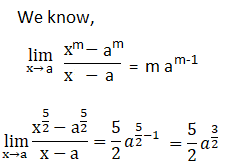Question 14: Evaluate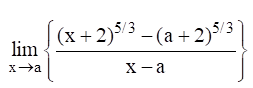Solution: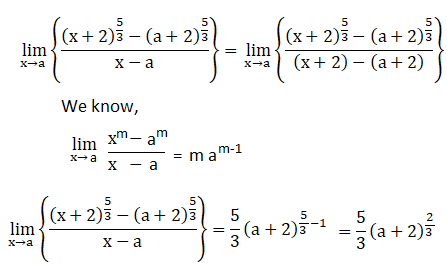Question 15: Evaluate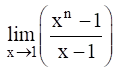Solution: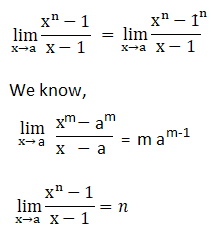Question 16: Evaluate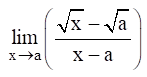Solution: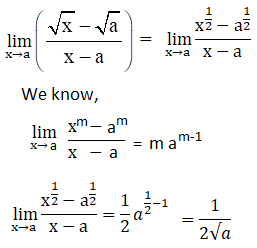Question 17: Evaluate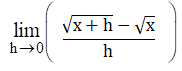Solution: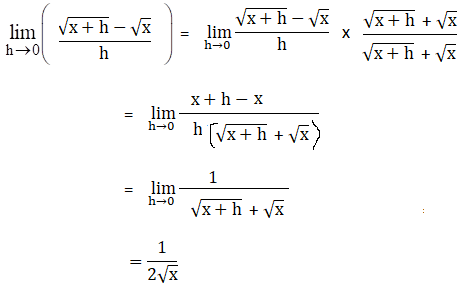Question 18: Evaluate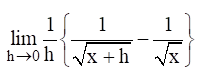Solution: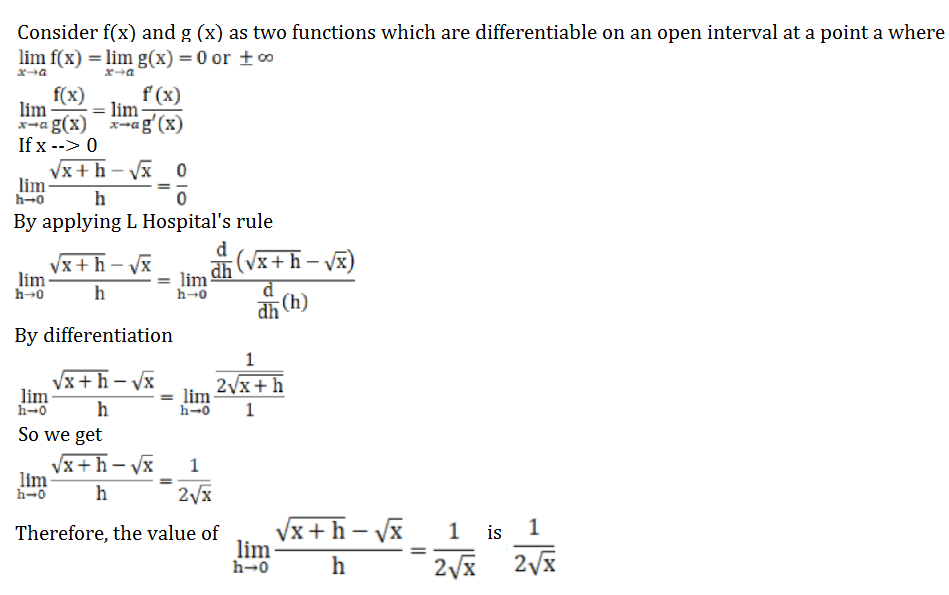Question 19: Evaluate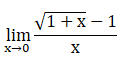Solution: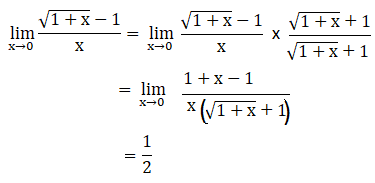Question 20: Evaluate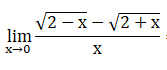Solution: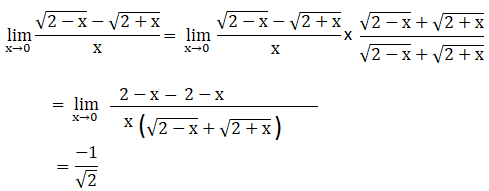## Access other exercise solutions of Class 11 Maths Chapter 27 Limits

Exercise 27B Solutions

Exercise 27C Solutions

## R S Aggarwal Solutions for Class 11 Maths Chapter 27 Exercise 27A

Class 11 Maths Chapter 27 Limits Exercise 27A is based on the following topics:

• Concepts of limit
• Rule for finding limits
• Fundamental theorems on limits